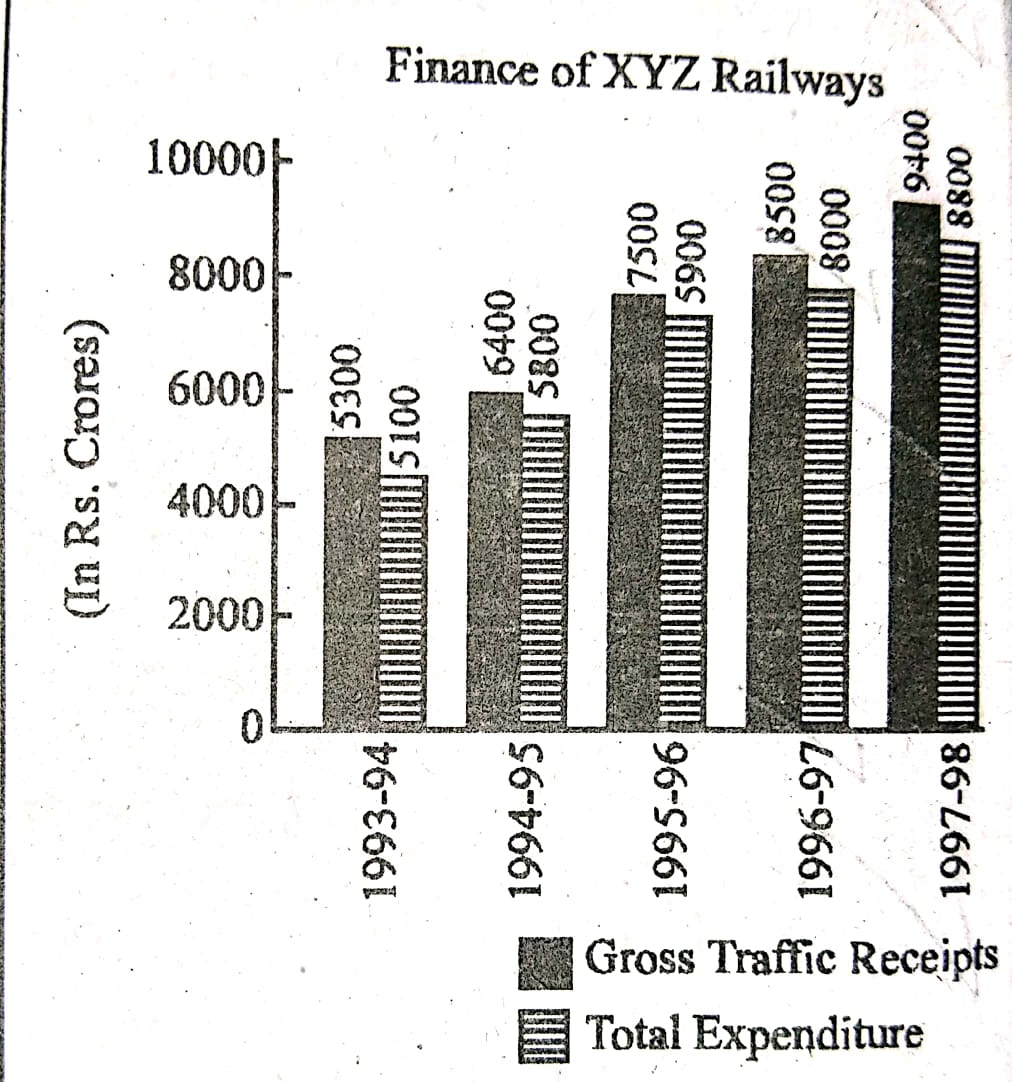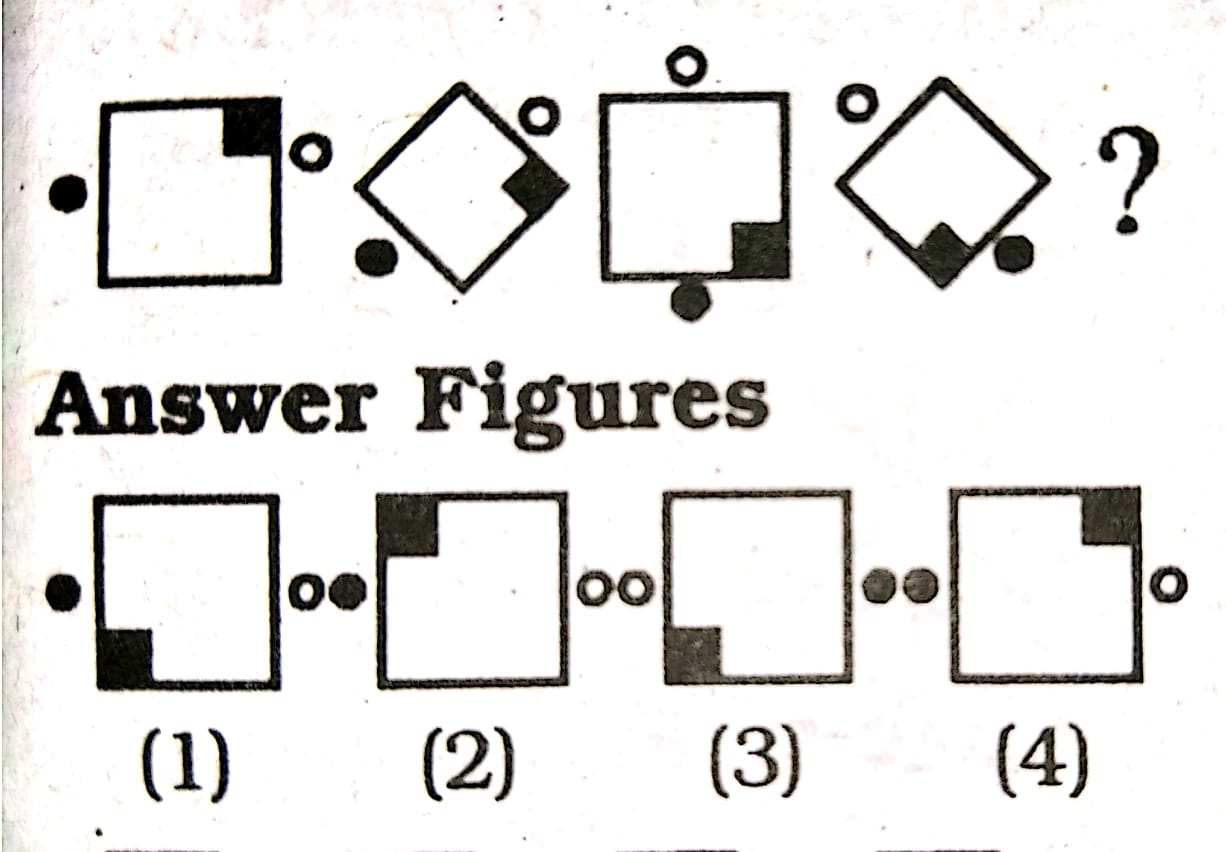HighDip.com
APTITUDE
 SELECT SHEET Sheet.No. 2019181716151413121110987654321

### SHEET. 20

 Q.197.1. What is the percentage increase in the gross traffic receipts in 1995-96 as compared to 1993-94? A. 33.9% B. 41.5% C. 20.7% D. 17% 2. If profit = gross traffic receipts - total expenditure, then in 1996-97, what percentage of gross traffic receipts is the profit made? A. 5.9% B. 6.4% C. 7.2% D. 8% 3. In which year was the profit as a percentage of gross traffic receipts the highest? A. 1997-98 B. 1996-97 C. 1995-96 D. 1994-95 4.In order to make a profit of 10%. What should have been gross traffic receipts (in Rs. crores) in 1994-95, total expenditure remaining the same? A. 5,667 B. 5,876 C. 6,444 D. 7,667 5. By what amount (in Rs. crores) has the expenditure increased over the period 1993-94 to 1997-98? A. 4,100 B. 3,900 C. 3,850 D. 3,700

 Q.196. Complete the series of figures, by selecting correct answer figure from the given responsesQ.195. A survey of film watching habits of people living in 5 cities, I, II, III, IV and V is summarized below. The column (1) gives percentage of film watchers in each city who see only one film a week. The column (2) gives the total number of film-watchers who see more than one film per week. Read the table and answer the following questions.

 City (1) (2) I 50 3200 II 30 3500 III 65 7000 IV 25 5400 V 80 2900

1. How many film-watchers in city II see only one film a week?
A. 900
B. 1500
C. 1600
D. 3200

2. Which city has the highest number of film-watchers who see only one film a week?
A. I
B. II
C. III
D. IV

3. The city with the lowest numbers of film-watchers is?
A. I
B. II
C. IV
D. V

4. The city with the highest numbers of film-watchers is?
A. I
B. V
C. IV
D. III

5. The total number of film-watchers in the five cities who see only one film a week is:
A. 31100
B. 29500
C. 28600
D. 28300

 Q.194. Assume that $\sqrt{13}=3.605 \text{ (Approx.)}$ $\sqrt{130}=11.40 \text{ (Approx.)}$ Find the value of $\sqrt{1.3}+\sqrt{1300}+\sqrt{0.013}$ A. 36.164 B. 37.304 C. 36.304 D. 37.164

 Q.193. Simplify : $1 + \frac{1}{1+\frac{2}{2+\frac{3}{1+\frac{4}{5}}}}$ A. $1\frac{11}{17}$ B. $1\frac{5}{7}$ C. $1\frac{6}{17}$ D. $1\frac{12}{17}$

 Q.192. In covering a certain distance, the speeds of A and B are in the ratio of 3:4. A takes 30 minutes more than B to reach the destination. The time taken by A to reach the destination is: A. $1$ hour B. $1\frac{1}{2}$ hours C. $2$ hours D. $2\frac{1}{2}$ hours

 Q.191. The distance between two cities A and B is 330 km. A train starts from A at 8 a.m. and travels towards B at 60 km/hr. Another train starts from B at 9 a.m. and travels towards A at 75 km/hr. At what time do they meet? A. 10 a.m. B. 10:30 a.m. C. 11 a.m. D. 11:30 a.m.